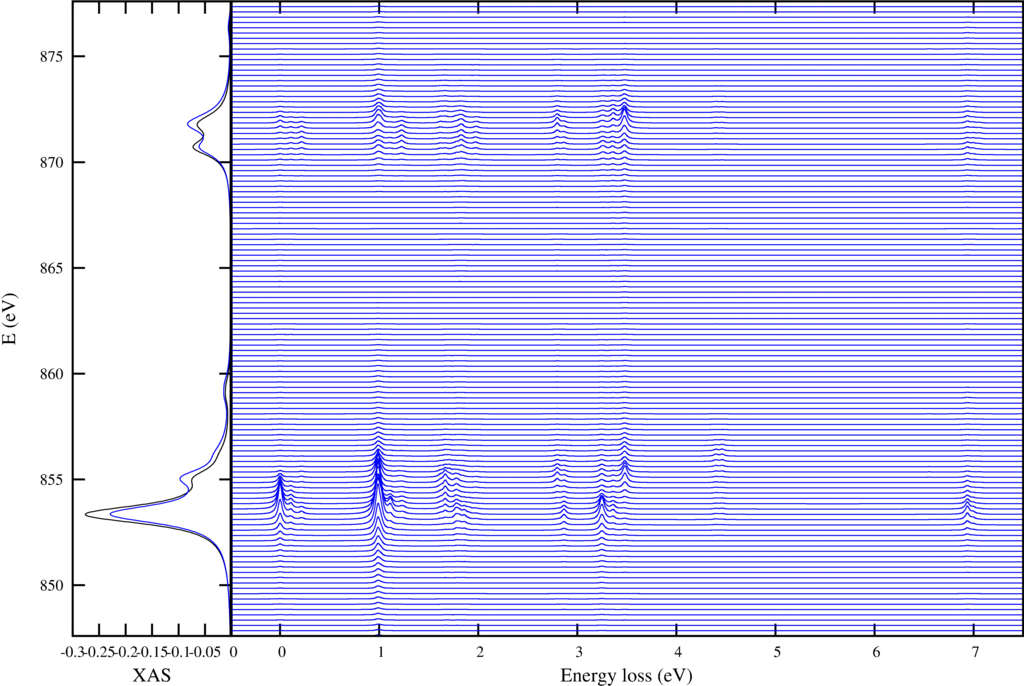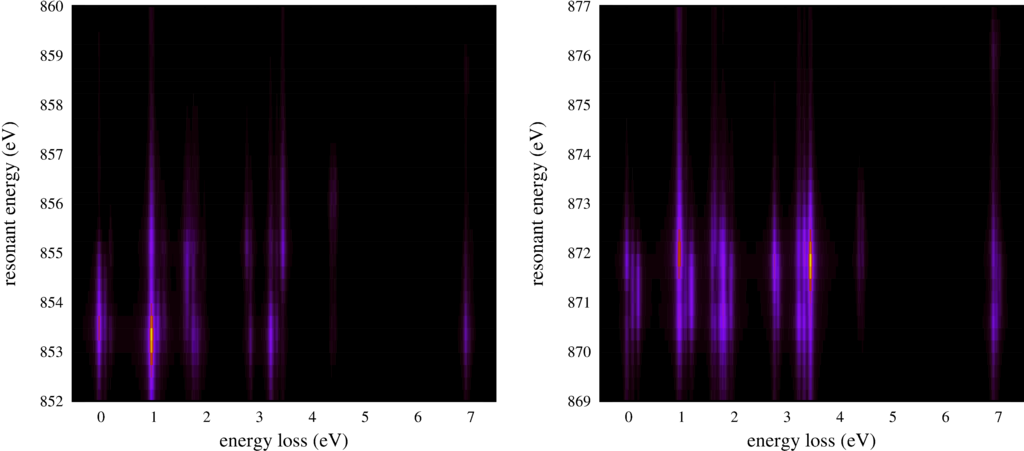# RIXS $L_{2,3}M_{4,5}$

Using the function ResonantSpectra we can calculate inelastic x-ray scattering. (or other second order processess) Here we show the example of $d$-$d$ excitations in NiO using RIXS. Due to an ever increasing resolution this method gains rapidly in popularity and impact. See for example the combined work of Ghiringhelli \textit{et al.} For NiO see \cite{Ghiringhelli:2005kp}.

A script to calculate these spectra is:

RIXS_L23M45.Quanty
-- this example calculates the resonant inelastic x-ray scattering in NiO we look at the
-- Ni L23M45 edge, i.e. we make an excitation from 2p to 3d (L23) and decay from the
-- 3d shell back to the 2p shell (final "hole" in the 3d shell M45 edge). These spectra
-- measure d-d excitations and or magnons.

-- We use the definitions of all operators and basis orbitals as defined in the file
-- 44_50_include and can afterwards directly continue by creating the Hamiltonian
-- and calculating the spectra

dofile("Include.Quanty")

-- The parameters and scheme needed is the same as the one used for XAS

-- We follow the energy definitions as introduced in the group of G.A. Sawatzky (Groningen)
-- J. Zaanen, G.A. Sawatzky, and J.W. Allen PRL 55, 418 (1985)
-- for parameters of specific materials see
-- A.E. Bockquet et al. PRB 55, 1161 (1996)
-- After some initial discussion the energies U and Delta refer to the center of a configuration
-- The L^10 d^n   configuration has an energy 0
-- The L^9  d^n+1 configuration has an energy Delta
-- The L^8  d^n+2 configuration has an energy 2*Delta+Udd
--
-- If we relate this to the onsite energy of the L and d orbitals we find
-- 10 eL +  n    ed + n(n-1)     U/2 == 0
--  9 eL + (n+1) ed + (n+1)n     U/2 == Delta
--  8 eL + (n+2) ed + (n+1)(n+2) U/2 == 2*Delta+U
-- 3 equations with 2 unknowns, but with interdependence yield:
-- ed = (10*Delta-nd*(19+nd)*U/2)/(10+nd)
-- eL = nd*((1+nd)*Udd/2-Delta)/(10+nd)
--
-- For the final state we/they defined
-- The 2p^5 L^10 d^n+1 configuration has an energy 0
-- The 2p^5 L^9  d^n+2 configuration has an energy Delta + Udd - Upd
-- The 2p^5 L^8  d^n+3 configuration has an energy 2*Delta + 3*Udd - 2*Upd
--
-- If we relate this to the onsite energy of the p and d orbitals we find
-- 6 ep + 10 eL +  n    ed + n(n-1)     Udd/2 + 6 n     Upd == 0
-- 6 ep +  9 eL + (n+1) ed + (n+1)n     Udd/2 + 6 (n+1) Upd == Delta
-- 6 ep +  8 eL + (n+2) ed + (n+1)(n+2) Udd/2 + 6 (n+2) Upd == 2*Delta+Udd
-- 5 ep + 10 eL + (n+1) ed + (n+1)(n)   Udd/2 + 5 (n+1) Upd == 0
-- 5 ep +  9 eL + (n+2) ed + (n+2)(n+1) Udd/2 + 5 (n+2) Upd == Delta+Udd-Upd
-- 5 ep +  8 eL + (n+3) ed + (n+3)(n+2) Udd/2 + 5 (n+3) Upd == 2*Delta+3*Udd-2*Upd
-- 6 equations with 3 unknowns, but with interdependence yield:
-- epfinal = (10*Delta + (1+nd)*(nd*Udd/2-(10+nd)*Upd) / (16+nd)
-- edfinal = (10*Delta - nd*(31+nd)*Udd/2-90*Upd) / (16+nd)
-- eLfinal = ((1+nd)*(nd*Udd/2+6*Upd)-(6+nd)*Delta) / (16+nd)
--
--
--
-- note that ed-ep = Delta - nd * U and not Delta
-- note furthermore that ep and ed here are defined for the onsite energy if the system had
-- locally nd electrons in the d-shell. In DFT or Hartree Fock the d occupation is in the end not
-- nd and thus the onsite energy of the Kohn-Sham orbitals is not equal to ep and ed in model
-- calculations.
--
-- note furthermore that ep and eL actually should be different for most systems. We happily ignore this fact
--
-- We normally take U and Delta as experimentally determined parameters

-- number of electrons (formal valence)
nd = 8
-- parameters from experiment (core level PES)
Udd     =  7.3
Upd     =  8.5
Delta   =  4.7
-- parameters obtained from DFT (PRB 85, 165113 (2012))
F2dd    = 11.14
F4dd    =  6.87
F2pd    =  6.67
G1pd    =  4.92
G3pd    =  2.80
tenDq   =  0.56
tenDqL  =  1.44
Veg     =  2.06
Vt2g    =  1.21
zeta_3d =  0.081
zeta_2p = 11.51
Bz      =  0.000001
H112    =  0.120

ed      = (10*Delta-nd*(19+nd)*Udd/2)/(10+nd)
eL      = nd*((1+nd)*Udd/2-Delta)/(10+nd)

epfinal = (10*Delta + (1+nd)*(nd*Udd/2-(10+nd)*Upd)) / (16+nd)
edfinal = (10*Delta - nd*(31+nd)*Udd/2-90*Upd) / (16+nd)
eLfinal = ((1+nd)*(nd*Udd/2+6*Upd) - (6+nd)*Delta) / (16+nd)

F0dd    = Udd + (F2dd+F4dd) * 2/63
F0pd    = Upd + (1/15)*G1pd + (3/70)*G3pd

Hamiltonian =  F0dd*OppF0_3d + F2dd*OppF2_3d + F4dd*OppF4_3d + zeta_3d*Oppldots_3d + Bz*(2*OppSz_3d + OppLz_3d) + H112 * (OppSx_3d+OppSy_3d+2*OppSz_3d)/sqrt(6) + tenDq*OpptenDq_3d + tenDqL*OpptenDq_Ld + Veg * OppVeg + Vt2g * OppVt2g + ed * OppN_3d + eL * OppN_Ld

XASHamiltonian =  F0dd*OppF0_3d + F2dd*OppF2_3d + F4dd*OppF4_3d + zeta_3d*Oppldots_3d + Bz*(2*OppSz_3d + OppLz_3d)+ H112 * (OppSx_3d+OppSy_3d+2*OppSz_3d)/sqrt(6) + tenDq*OpptenDq_3d + tenDqL*OpptenDq_Ld + Veg * OppVeg + Vt2g * OppVt2g + edfinal * OppN_3d + eLfinal * OppN_Ld + epfinal * OppN_2p + zeta_2p * Oppcldots + F0pd * OppUpdF0 + F2pd * OppUpdF2 + G1pd * OppUpdG1 + G3pd * OppUpdG3

-- we now can create the lowest Npsi eigenstates:
Npsi=3
-- in order to make sure we have a filling of 8 electrons we need to define some restrictions
StartRestrictions = {NF, NB, {"000000 00 1111111111 0000000000",8,8}, {"111111 11 0000000000 1111111111",18,18}}

psiList = Eigensystem(Hamiltonian, StartRestrictions, Npsi)
oppList={Hamiltonian, OppSsqr, OppLsqr, OppJsqr, OppSx_3d, OppLx_3d, OppSy_3d, OppLy_3d, OppSz_3d, OppLz_3d, Oppldots_3d, OppF2_3d, OppF4_3d, OppNeg_3d, OppNt2g_3d, OppNeg_Ld, OppNt2g_Ld, OppN_3d}

-- print of some expectation values
print("  #    <E>      <S^2>    <L^2>    <J^2>    <S_x^3d> <L_x^3d> <S_y^3d> <L_y^3d> <S_z^3d> <L_z^3d> <l.s>    <F>   <F>   <Neg^3d> <Nt2g^3d><Neg^Ld> <Nt2g^Ld><N^3d>");
for i = 1,#psiList do
io.write(string.format("%3i ",i))
for j = 1,#oppList do
expectationvalue = Chop(psiList[i]*oppList[j]*psiList[i])
io.write(string.format("%8.3f ",expectationvalue))
end
io.write("\n")
end

-- Not the main task of this example, but in order to know where the resonance sits it is
-- nice to calculate the x-ray absorption spectra
XASSpectra = CreateSpectra(XASHamiltonian, T2p3dx, psiList, {{"Emin",-10}, {"Emax",20}, {"NE",3500}, {"Gamma",1.0}})
XASSpectra.Print({{"file", "RIXSL23M45_XAS.dat"}})

-- We also calculate the fluorescence yield spectra which are equal to the integral over the
-- RIXS spectra
FYSpectra = CreateFluorescenceYield(XASHamiltonian, T2p3dx, T3d2py, psiList, {{"Emin",-10}, {"Emax",20}, {"NE",3500}, {"Gamma",1.0}})
FYSpectra.Print({{"file","RIXSL23M45_FY.dat"}})

-- and we calculate the RIXS spectra. Note that in order to calculate RIXS you need to
-- specify two Hamiltonians.
-- These calculations can be lengthy and one should think that for each incoming energy
-- (E1) one needs to do a new calculation. In this case there are thus 120 calculations
RIXSSpectra = CreateResonantSpectra(XASHamiltonian, Hamiltonian, T2p3dx, T3d2py, psiList, {{"Emin1",-10}, {"Emax1",20}, {"NE1",120}, {"Gamma1",1.0}, {"Emin2",-0.5}, {"Emax2",7.5}, {"NE2",8000}, {"Gamma2",0.05}})
RIXSSpectra.Print({{"file", "RIXSL23M45.dat"}})

print("Finished calculating the spectra now start plotting.\nThis might take more time than the calculation");

-- Once finished we can make some nice plots. The spectra are saved to disk in ASCII format
-- but I like to add gnuplot scripts so you can look at pictures imeidately
gnuplotInput = [[
set autoscale
set xtic auto
set ytic auto
set style line  1 lt 1 lw 1 lc rgb "#000000"
set style line  2 lt 1 lw 1 lc rgb "#FF0000"
set style line  3 lt 1 lw 1 lc rgb "#00FF00"
set style line  4 lt 1 lw 1 lc rgb "#0000FF"

set out 'RIXSL23M45_Map.ps'
set terminal postscript portrait enhanced color  "Times" 8 size 7.5,6

unset colorbox

energyshift=857.6

set multiplot
set size 0.5,0.55
set origin 0,0

set ylabel "resonant energy (eV)" font "Times,10"
set xlabel "energy loss (eV)" font "Times,10"

set yrange [852:860]
set xrange [-0.5:7.5]

plot "<(awk '((NR>5)&&(NR<8007)){for(i=3;i<=NF;i=i+2){printf \"%s \", $i}{printf \"%s\", RS}}' RIXSL23M45.dat)" matrix using ($2/1000-0.5):($1/4+energyshift-10):(-$3) with image notitle

set origin 0.5,0

set yrange [869:877]
set xrange [-0.5:7.5]

plot "<(awk '((NR>5)&&(NR<8007)){for(i=3;i<=NF;i=i+2){printf \"%s \", $i}{printf \"%s\", RS}}' RIXSL23M45.dat)" matrix using ($2/1000-0.5):($1/4+energyshift-10):(-$3) with image notitle

unset multiplot

set out 'RIXSL23M45_Spec.ps'
set terminal postscript portrait enhanced color  "Times" 8 size 7.5,5

set multiplot
set size 0.25,1.0
set origin 0,0

set ylabel "E (eV)" font "Times,10"
set xlabel "XAS" font "Times,10"
set yrange [energyshift-10:energyshift+20]
set xrange [-0.3:0]
plot "RIXSL23M45_XAS.dat"  using       3:($1+energyshift) notitle with lines ls 1,\ "RIXSL23M45_FY.dat" using (-3*$2):($1+energyshift) notitle with lines ls 4 set size 0.8,1.0 set origin 0.2,0.0 set xlabel "Energy loss (eV)" font "Times,10" unset ylabel unset ytics set xrange [-0.5:7.5] ofset = 0.25 scale=3 plot for [i=0:120] "RIXSL23M45.dat" using 1:(-column(3+2*i)*scale+ofset*i-10 + energyshift) notitle with lines ls 4 unset multiplot ]] -- write the gnuplot script to a file file = io.open("RIXSL23M45.gnuplot", "w") file:write(gnuplotInput) file:close() -- call gnuplot to execute the script os.execute("gnuplot RIXSL23M45.gnuplot") -- transform to pdf and eps os.execute("ps2pdf RIXSL23M45_Map.ps ; ps2eps RIXSL23M45_Map.ps ; mv RIXSL23M45_Map.eps temp.eps ; eps2eps temp.eps RIXSL23M45_Map.eps ; rm temp.eps") os.execute("ps2pdf RIXSL23M45_Spec.ps ; ps2eps RIXSL23M45_Spec.ps ; mv RIXSL23M45_Spec.eps temp.eps ; eps2eps temp.eps RIXSL23M45_Spec.eps ; rm temp.eps") It produces two plots.  Resonant inelastic x-ray scattering spectra for different incoming and outgoing photon energies.The first shows on the left the XAS spectra in black and the integrated RIXS spectra (FY) in blue. The resonant enhanced$d$-$d\$ excitations are shown in the right plot.

 Resonant inelastic x-ray scattering spectra for different incoming and outgoing photon energies.The second plot shows the same RIXS spectra, but now as an intensity map.

The text output of the script is:

RIXS_L23M45.out
  #    <E>      <S^2>    <L^2>    <J^2>    <S_x^3d> <L_x^3d> <S_y^3d> <L_y^3d> <S_z^3d> <L_z^3d> <l.s>    <F>   <F>   <Neg^3d> <Nt2g^3d><Neg^Ld> <Nt2g^Ld><N^3d>
1   -3.503    1.999   12.000   15.095   -0.370   -0.115   -0.370   -0.115   -0.741   -0.230   -0.305   -1.042   -0.924    2.186    5.990    3.825    6.000    8.175
2   -3.395    1.999   12.000   15.160   -0.002   -0.000   -0.002   -0.000   -0.003   -0.001   -0.322   -1.043   -0.925    2.189    5.988    3.823    6.000    8.178
3   -3.286    1.999   12.000   15.211    0.369    0.113    0.369    0.113    0.737    0.227   -0.336   -1.043   -0.925    2.193    5.987    3.820    6.000    8.180
Start of LanczosTriDiagonalizeKrylovMC
Start of LanczosTriDiagonalizeKrylovMC
Finished calculating the spectra now start plotting.
This might take more time than the calculation

Workshops

Script versionsExternal programsPrivacy

##### Tools